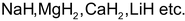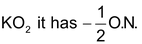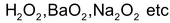# Oxidation Number Or Oxidation State

## Oxidation Number Or Oxidation State

Oxidation no. of an element may be defined as the charge that an atom of the element has in its ion or appears to have when present in the combined states with other atoms

Rules for assigning oxidation number

The O.N of the element in the free or elementary state is always zero irrespective of its allotropic form

Oxidation number of helium is He = 0

Oxidation number of chlorine is Cl2 = 0

Oxidation number of sulphur in S8 = 0

Oxidation number of phosphorus in P4 = 0

• The O. N. of the element in the monoatomic ion is equal to the charge on the ion. For example, in K+Cl-, the O.N. of K is +1 while that Cl is –1
• O.N. of all alkali metals is +1 while those of alkaline earth metals is +2 in all their compounds
• The O.N. of fluorine is always –1 in all its compounds. (Note that fluorine is a most electronegative element, hence can attain positive oxidation state)
• Hydrogen is assigned +1 O.N. when it is attached to more electronegative element but it has –1 O.N. in metal hydrides like• Oxygen is assigned O.N –2 in most of its compounds.

Exceptions: F2O, hence O is in +2 oxidation state.

In super oxides likeIn peroxides likeO.N is –1.

In ozonide, the oxidation number of oxygen is assigned −1/3.

• By the principle of conservation of charge, the algebraic sum of the oxidation number of all the atoms in a molecule is zero. But in case of polyatomic ion the sum of O.N. of all its atoms is equal to charge on the ion.
• The maximum O.N. of an element can be equal to its group number. (Except O and F)
• Minimum O.N. of an element can be equal to (8-n) n is the group no. of the element (Except metals)
• The evaluation of O.N cannot be made directly in some cases e.g. HCN by using the above rule since we have no rule for oxidation No. of both N and C.

In all such cases evaluation of O.N should be made be taking contribution of covalent bonds and coordinate bonds

1. When two atoms are attached with the help of a single covalent bond then its contribution for a less electronegative atom is +1 and for a more electronegative atom is –1.
2. When a covalent bond is present between the two same atoms then its contribution will be zero for both the atoms
3. In the case of the Coordinate bond, it gives a +2 value of oxidation number to a less electronegative atom and -2 values to a more electronegative atom when the coordinate bond is directed from a less electronegative atom to a more electronegative atom.
4. If a coordinate bond is directed from a more electronegative to a less electronegative atom then its contribution will be zero for both atoms.